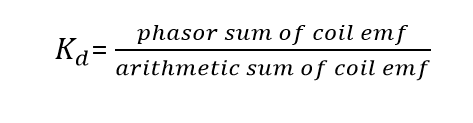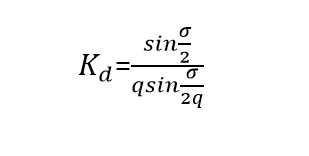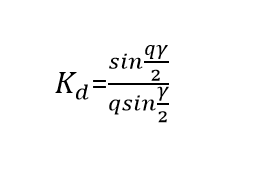# What is Distribution factor?

### Distribution Factor

The distribution factor is defined as the ratio of phasor sum of coil emf to the arithmetic sum of coil emf which is denoted as Kd. It is also known as belt factor or breadth factor. The formula for finding Distribution factor is,Note that, Distribution factor is always less than unity.Where σ = q x γ

γ = slot angular pitch

q = slot per pole per phase

Hence,### Why does Distribution factor need to be calculated?

Generally, Concentrated winding is only used where a number of slots on the armature is equal to the number of poles. This type of winding gives maximum output voltage but not exactly sinusoidal. For obtaining smooth sinusoidal waveform we use distributed winding. The output voltage is less as compared to concentrated winding but which has many Advantages

1. In reduces the harmonics present in the generated emf so improved sine wave will have obtained.
2. The conductor is distributed over the slots so the core (iron and copper) is fully utilized.
3. It also improves the mechanical strength of the winding.